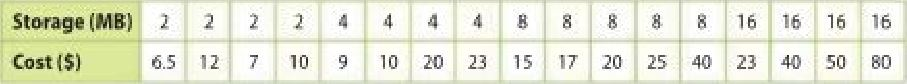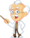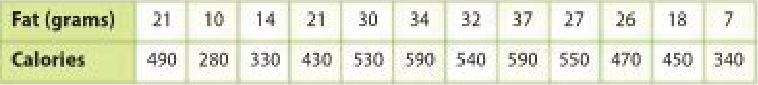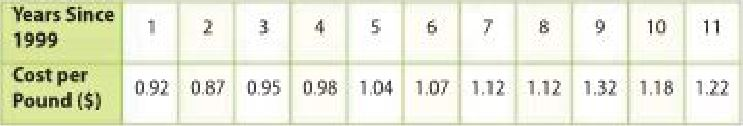Homework Explained - Math Practice 101Dear guest, you are not a registered member. As a guest, you only have read-only access to our books, tests and other practice materials.

As a registered member you can:

 Extra Practice   Copy and Solve For Exercises 8-14, show your work and answers on a separate piece of paper. Question 8 The table shows the storage amount and cost for flash drives at a local electronics store.a. Construct a scatter plot for the data. Then draw and assess a line that best represents the data. Type below:(show solution) Question 8 b. Write an equation in slope-intercept form for the line of fit and interpret the slope and y-intercept. Type below:(show solution) Question 8 c. Use the equation to make a conjecture about the cost of a flash drive with a storage capacity of 32 MB. Type below:(show solution) Question 9 Model with Mathematics The table shows fat and Calories for fast food sandwiches.a. Construct a scatter plot for the data. Draw and assess a line that best represents the data. Type below:(show solution) Question 9 b. Write an equation in slope-intercept form for the line of fit and interpret the slope and y-intercept. Type below:(show solution) Question 9 c. Use the equation to make a conjecture about the number of grams of fat in a sandwich with 350 Calories. Type below:(show solution) Question 10 The table shows the cost per pound of apples for several years.a. Construct a scatter plot for the data. Draw and assess a line that best represents the data. Type below:(show solution) Question 10 b. Write an equation in slope-intercept form for the line of fit and interpret the slope and y-intercept. Type below:(show solution) Question 10 c. Use the equation to make a conjecture about the cost of apples in the year 2025. Type below:(show solution)